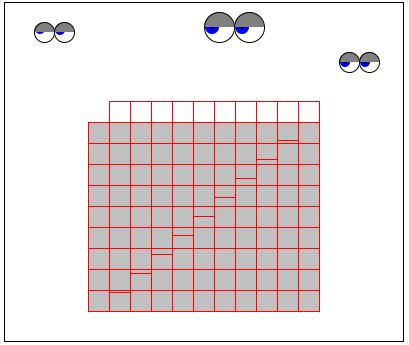# The Eye Opener Series

It's said that a picture is worth a thousand words. By implication, an interactive, animated picture is worth ... eh, at least that much. Eye Opener Series is a collection of Java applets that illustrate and thus help solve or prove various math problems. Below is one such applet. It's interesting to note that the total of Java code that constitutes this applet is 10K. Given its size of 400x350 pixels, a comparable .gif file may easily take as much .

Now, the gray rectangle below is cut into two pieces (upper and lower) by a staircase line. You can drag the upper piece one square up and to the right. Please do this. Try to fit the upper portion into its new position.

### If you are reading this, your browser is not set to run Java applets. Try IE11 or Safari and declare the site https://www.cut-the-knot.org as trusted in the Java setup.I hope that by now you may have gotten a hunch why I call this applet an Eye Opener. You may legitimately ask what is this the applet helps to solve. The answer is simple.

99 = 100

Indeed, You start with a gray rectangle whose area is 9 squares by 11, that is, 99. At the end of the exercise, the rectangle gets reconfigured into a square 10×10 which is 100. In the upper right corner of the applet three controls let you select a different configuration. Changing it one can easily prove additional identities - 24 = 25, 48 = 49, etc.

(Darryl Atkinson has jokingly remarked that "if you move the bunch of squares down and left instead of up and right, you find that there are now 98 squares. Therefore 98 = 99 = 100." I may add that, as a matter of fact, from 99 = 100 follows anything that may cross one's mind - all in a very logical fashion. Still, it's nice to have a geometric support for an overlooked fact :)

Ok, it's clear that some cheating is involved some place. Try to figure out where. Regardless, there is a positive experience to acquire. Mathematics in general and Geometry in particular are deductive sciences. Paper or even a computer may serve as tools and illustrations that help solve problems. However, nothing can replace a rigorous proof.

Allowing for a pun, I'll try to illustrate the latter point using the applets from the Eye Opener Series.

Just to end the introduction and, perhaps, to confuse things a little, I do believe that the applet's picture does prove something. Perhaps a better way to put it is to say that it helps visualize a proof of the following identity

(n-1)×(n+1) = n2-1

Indeed, generally speaking the gray rectangle's area is (for a given n) (n-1)×(n+1). The area of the final square is n2. The upper row of the square is the one that does not fit into the rectangle. On the other hand, the leftmost column of the rectangle is the one that does not fit into the square. Now, the row contains n small squares whereas the column contains only n-1 small squares. Which proves that the area of the square exceeds that of the rectangle by one small square.

Trying to use a picture as the main tool in my proof, do you think I contradict myself? I do not believe I do. Mathematics is very graphical and draws heavily on human imagination and ability to visualize. As long as you know exactly without measuring the rectangles that there are sides of 10 or 11 units a drawing will furnish a legitimate support tool that one can do actually without. For example, the above can be formalized in the following manner.

N1 = (n-1)×(n+1) is the area of the original rectangle
N2 = n×n is the are of the square

Now, let's split the rectangle into the first column and the remaining rectangle. Let's also split the square into the upper row and the remaining rectangle: (by the distributive law)

N1 = (n-1) + (n-1)n and
N2 = n + n(n-1)

Thus we see that N2 - N1 = [n + n(n-1)] - [(n-1) + (n-1)n] = 1. Q.E.D.

There is another example of how geometry helps visualize algebraic proofs.Now, to the rest of the series. Oh, a word of warning. All of the applets depend on a mouseTracker.class - a piece of Java code that controls a couple of eyes. Due to some idiosyncrasy of implementation, after you run several of this applets, the browser (I used Netscape 2 and 3 for Windows 95) refuses to load this class. Must be a bug... I am sure they'll fix it in later releases. Meanwhile, the only solution I found was to exit the browser and then restart it again.